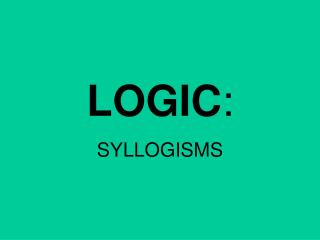DownloadDownload PresentationLOGIC :

LOGIC :

Télécharger la présentationLOGIC :

- - - - - - - - - - - - - - - - - - - - - - - - - - - E N D - - - - - - - - - - - - - - - - - - - - - - - - - - -
Presentation Transcript

1. LOGIC: SYLLOGISMS

2. Syllogism: A set of statements called premises, which lead to one logical conclusion.

3. If the premises are true, (and we assume they are), then the conclusion must be true.

4. (Note: This symbol means “Therefore”. It signals the conclusion.)

5. Law of Syllogism

6. If a figure is a rectangle, then it is a parallelogram. If a figure is a parallelogram, then its diagonals bisect each other. __________________________

7. If a figure is a rectangle, then it is a parallelogram. If a figure is a parallelogram, then its diagonals bisect each other. __________________________

8. If a figure is a rectangle, then it is a parallelogram. If a figure is a parallelogram, then its diagonals bisect each other. __________________________

9. If a figure is a rectangle, then it is a parallelogram. If a figure is a parallelogram, thenits diagonals bisect. __________________________ If a figure is a rectangle, then its diagonals bisect.

10. Law of Detachment

11. If a figure is a rectangle, then it is a parallelogram. ABCD is a rectangle. __________________________

12. If a figure is a rectangle, then it is a parallelogram. ABCD is a rectangle. __________________________

13. If a figure is a rectangle, then it is a parallelogram. ABCD is a rectangle. __________________________ ABCD is a parallelogram.

14. Law of Contrapositive

15. If a figure is a rectangle, then it is a parallelogram. ABCD is not a parallelogram. __________________________

16. If a figure is a rectangle, then it is a parallelogram. ABCD is not a parallelogram. __________________________

17. If a figure is a rectangle, then it is a parallelogram. ABCD is not a parallelogram. __________________________ ABCD is not a rectangle.

18. Time to Practice:

19. In the following examples, use the given law of syllogism to draw the correct conclusion from the set of premises.

20. 1. If frogs fly then toads talk. Frogs fly. -----------------------------

21. Law of Detachment

22. 1. If frogs fly then toads talk. Frogs fly. -----------------------------

23. 1. If frogs fly then toads talk. Frogs fly. ----------------------------- Toads talk.

24. 2. If hens heckle then crows don’t care. Crows care. -----------------------

25. Law of Contrapositive

26. 2. If hens heckle then crows don’t care. Crows care. -----------------------

27. 2. If hens heckle then crows don’t care. Crows care. ----------------------- Hens don’t heckle.

28. 3. If ants don’t ask then flies don’t fret. Ants don’t ask. ----------------------------

29. Law of Detachment

30. 3. If ants don’t ask then flies don’t fret. Ants don’t ask. ----------------------------

31. 3. If ants don’t ask then flies don’t fret. Ants don’t ask. ---------------------------- Flies don’t fret.

32. 4. If Dave dives then Cal caves. If Cal caves then Hank hikes. -------------------------------------

33. Law of Syllogism

34. 4. If Dave dives then Cal caves. If Cal caves then Hank hikes. -------------------------------------

35. 4. If Dave dives then Cal caves. If Cal caves then Hank hikes. ------------------------------------- If Dave dives then Hank hikes.

36. 5. If dogs duel then rats wrestle. Rats don’t wrestle. -----------------------------

37. Law of Contrapositive

38. 5. If dogs duel then rats wrestle. Rats don’t wrestle. -----------------------------

39. 5. If dogs duel then rats wrestle. Rats don’t wrestle. ----------------------------- Dogs don’t duel.

40. Why does this one work?

41. Here are the premises:

42. Replace with contrapositive:

43. Replace with contrapositive:

44. Use Law of Detatchment:

45. Law of Contrapositive